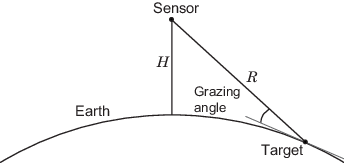# grazingang

Grazing angle of surface target

## Syntax

```grazAng = grazingang(H,R) grazAng = grazingang(H,R,MODEL) grazAng = grazingang(H,R,MODEL,Re) grazAng = grazAng = grazingang(___,'TargetHeight',TGTHT) ```

## Description

`grazAng = grazingang(H,R)` returns the grazing angle for a sensor `H` meters above the surface, to surface targets `R` meters away. The computation assumes a curved earth model with an effective earth radius of approximately 4/3 times the actual earth radius.

`grazAng = grazingang(H,R,MODEL)` also specifies the earth model used to compute the grazing angle. `MODEL` is either `'Flat'` or `'Curved'`.

`grazAng = grazingang(H,R,MODEL,Re)` also specifies the effective earth radius. Effective earth radius applies to a curved earth model. When `MODEL` is `'Flat'`, the function ignores `Re`.

`grazAng = grazAng = grazingang(___,'TargetHeight',TGTHT)` also specifies the target height, `TGTHT` above the surface as either a scalar or a vector. If any combination of `ht`, `R`, and `TGTHT` are vectors, then the dimensions must be equal. `R` must be greater than or equal to the absolute value of the difference of `HT` and `TGTHT`.

## Input Arguments

 `H` Height of the sensor above the surface, in meters. This argument can be a scalar or a vector. If both `H` and `R` are nonscalar, they must have the same dimensions. `R` Distance in meters from the sensor to the surface target. This argument can be a scalar or a vector. If both `H` and `R` are nonscalar, they must have the same dimensions. `R` must be between `H` and the horizon range determined by `TGTHT`. `MODEL` Earth model, as one of | `'Curved'` | `'Flat'` |. Default: `'Curved'` `Re` Effective earth radius in meters. This argument must be a positive scalar value. You can used `effearthradius` to compute the effective radius. Default: `effearthradius`, which is approximately 4/3 times the actual earth radius `TGTHT` Target height above surface, specified as a scalar or vector. If any combination of `H`, `R`, and `TGTHT` are vectors, then their sizes must be equal. `R` must be greater than or equal to the absolute value of the difference of `H` and `TGTHT`. A surface target has a `TGTHT` of zero. Default: `0`

## Output Arguments

 `grazAng` Grazing angle, in degrees. The size of `grazAng` is the larger of `size(H)` and `size(R)`.

## Examples

collapse all

Determine the grazing angle (in degrees) of a path to a ground target located 1.0 km from a sensor. The sensor is mounted on a platform that is 300 m above the ground.

`grazAng = grazingang(300,1.0e3)`
```grazAng = 17.4544 ```

collapse all

### Grazing Angle

The grazing angle is the angle between a line from the sensor to a surface target, and a tangent to the earth at the site of that target.For the curved earth model with an effective earth radius of Re, the grazing angle is:

`${\mathrm{sin}}^{-1}\left(\frac{{H}^{2}+2H{R}_{e}-{R}^{2}}{2R{R}_{e}}\right)$`

For the flat earth model, the grazing angle is:

`${\mathrm{sin}}^{-1}\left(\frac{H}{R}\right)$`

## References

 Long, Maurice W. Radar Reflectivity of Land and Sea, 3rd Ed. Boston: Artech House, 2001.

 Ward, J. “Space-Time Adaptive Processing for Airborne Radar Data Systems,” Technical Report 1015, MIT Lincoln Laboratory, December, 1994.

## Extended Capabilities